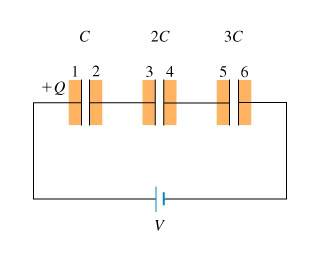# Capacitors in Series and finding voltage

We've been studying capacitors in series in my physics class. I have a problem tonight and I know the answer, I just don't know how to get to it.

The problems is as follows:Find the voltage V1 across the first capacitor.

ranger
Gold Member
capacitors in sereis always have the same charge. They do not the same voltage unless they have the same capacitance.

What would be your first step?

capacitors in sereis always have the same charge. They do not the same voltage unless they have the same capacitance.

What would be your first step?

The first step is finding that they all have the same charge. Then, I think, I would find the equivalent capacitance which is equal to 11/6 C. From there I don't know where I would get the voltage for each one.

ranger
Gold Member
Do you know also know of a formula that relates capacitance, voltage, and charge?

Do you know also know of a formula that relates capacitance, voltage, and charge?

ofcourse, c=q/v... So Ceq=Q/Veq...

ranger
Gold Member
ofcourse, c=q/v... So Ceq=Q/Veq...

Good. But instead of Ceq=Q/Veq (not sure what you mean by Veq). It should be Ceq=Q/V, where V is the total source voltage.

We know what Ceq and V is simply V, whats left to be determined? Remember that this quantity would be the same for all caps.

Good. But instead of Ceq=Q/Veq (not sure what you mean by Veq). It should be Ceq=Q/V, where V is the total source voltage.

We know what Ceq and V is simply V, whats left to be determined? Remember that this quantity would be the same for all caps.

Ok by the Veq I meant the total voltage... So what we have is that the total voltage is equal to V. The C equivalence is equal to 11/6 C. And that the charge on each capacitor is Q. Now from that information, I am still at a loss as to how to determine the voltage across the first capacitor.

The answer is 6/11 V according to the system my homework is in...

ranger
Gold Member
Are you sure the Ceq = (11C)/6? Recheck it. If the charge on each cap is Q, then what is Q? Knowing Q, you will be able to find V for any cap.

Are you sure the Ceq = (11C)/6? Recheck it. If the charge on each cap is Q, then what is Q? Knowing Q, you will be able to find V for any cap.

Ok, I see that I made the mistake there... It is 6/11 C. So we would then set that 6/11 C = Q(for each capacitor) / (Vtot).

So Q = 6 (Vtot) (C) / 11 for each capacitor.

Now that we have that, we could then find the voltage by knowing the q=v/c equation.

C = (6/11 C Vtot / 11)/V1
C V1 = 6/11 C Vtot
V1 = 6/11 Vtot

You're very much welcome. And well done on your part